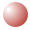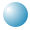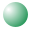math jokes 4 mathy folks
live. laugh. learn.

A Latin square is an n × n square in which n groups of n elements are divided so that there is one element from each group in every row and every column. For example, the figure to the left shows the (trivial) solution for a 2 × 2 square.

Arrange the 16 spheres onto the 4 × 4 square so that there is one sphere of each color in every row and every column. Click and drag the spheres to move them onto the grid.How many different arrangements can you find?

2 × 2 Latin SquareBoth rows and both columns contain one red and one blue sphere.

A normalized Latin square is one in which the order of the elements is the same in the top row and the left column.